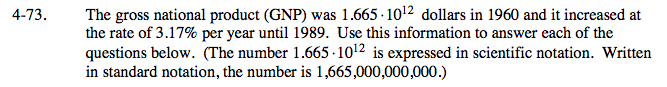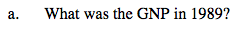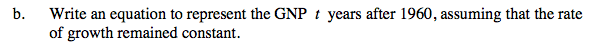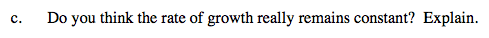### Home > A2C > Chapter 4 > Lesson 4.2.1 > Problem4-73

4-73.
1. The gross national product (GNP) was 1.665 · 1012 dollars in 1960 and it increased at the rate of 3.17% per year until 1989. Use this information to answer each of the questions below. (The number 1.665 · 1012 is expressed in scientific notation. Written in standard notation, the number is 1,665,000,000,000.) Homework Help ✎

1. What was the GNP in 1989?

2. Write an equation to represent the GNP t years after 1960, assuming that the rate of growth remained constant.

3. Do you think the rate of growth really remains constant? Explain.y = 1.665 · 1012(1.0317)29

Multiply the GNP in 1960 by 1.0317 twenty-nine times.

The GNP in 1989 is 4.116 · 1012 dollars.Remember that the GNP increases by 3.17% each year.

y = 1.66(1012)(1.0317)tIf you don’t know or you aren’t sure, try looking it up on the internet. Look for a graph. You could enter GNP USA into a search engine.